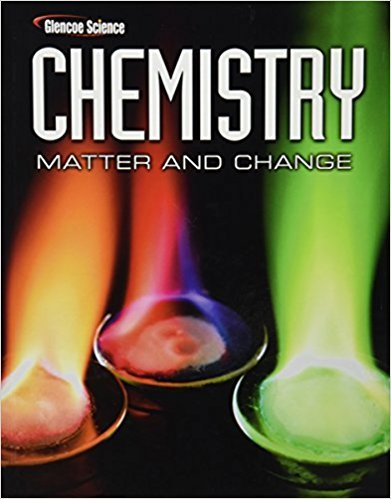### Solution Found!# Challenge 106.5 g of HCl(g) react with an unknown amount

Chapter , Problem 9

(choose chapter or problem)

Get Unlimited Answers! Check out our subscriptions
QUESTION:

Challenge 106.5 g of HCl(g) react with an unknown amount of N H 3(g) to produce 157.5 g of N H 4Cl(s). How many grams of N H 3(g) reacted? Is the law of conservation of mass observed in the reaction? Justify your answer.

QUESTION:

Challenge 106.5 g of HCl(g) react with an unknown amount of N H 3(g) to produce 157.5 g of N H 4Cl(s). How many grams of N H 3(g) reacted? Is the law of conservation of mass observed in the reaction? Justify your answer.

Problem 9

Challenge 106.5 g of HCl(g) react with an unknown amount ofto produce 157.5 g of. How many grams ofreacted? Is the law of conservation of mass observed in the reaction? Justify your answer.

Step by step solution

Step 1 of 2

From the given,

Produced weight of ammonium chloride = 157.5g

Weight of hydrogen chloride = 106.5 g